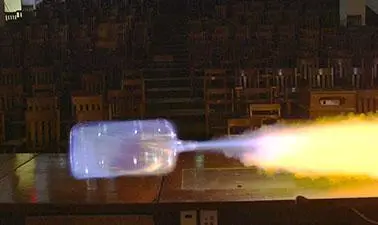There is 0 item now in your comparison listView a comparison list

# Mechanics, Part 1

Price
\$169
22,940 people completed this program

## Overview

This course is an introduction to mechanics and follows a standard first-semester university physics course. You will learn fundamental mechanics concepts and mathematical problem solving required for all STEM fields.

The course begins with kinematics, where you will learn to use equations and graphs to describe the position, velocity, and acceleration of an object, and how those quantities are related through calculus. You will then learn how forces affect motion through Newton’s Laws, and how to understand and calculate several different forces, including gravitational, normal force, drag force, and friction force. The concept of energy will be covered, including kinetic energy, potential energy, and how they are affected by work. You will learn how to use the conservation of energy to solve motion problems. Finally, momentum, another “quantity of motion” will be described. You will learn how to calculate momentum, about its relationship to Newton’s laws, and how to use it to solve motion problems, including collisions.

This course is valuable preparation for the equivalent on-campus course, or as supplementary material.

• Kinematics
• Work and Energy
• Momentum and Collisions
• Conservation laws
• How to apply vector analysis and calculus to solve physics problems

## Syllabus

Week 1: 1D Motion

Week 2: 2D Motion

Week 3: Newton’s Laws

Week 4: Energy

Week 5: Momentum and Collisions

Rice University

## Reviews(0)

Help others make their choice. Be the first one to leave a review

Leave a reviewBeginner
English
6 weeks
Self-paced
Online